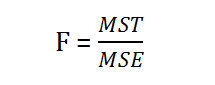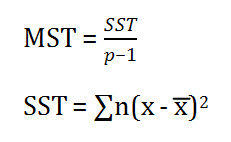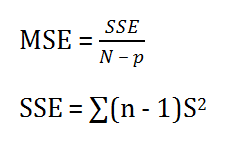5 minutes
share
Analysis of variance, or ANOVA, is a strong statistical technique that is used to show difference between two or more means or components through significance tests. It also shows us a way to make multiple comparisons of several population means. The Anova test is performed by comparing two types of variation, the variation between the sample means, as well as the variation within each of the samples. Below mentioned formula represents one way Anova test statistics:Where,
F = Anova Coefficient
MST = Mean sum of squares due to treatment
MSE = Mean sum of squares due to error
Formula for MST is given below:Where,
SST = Sum of squares due to treatment
p = Total number of populations
n = The total number of samples in a population
Formula for MSE is given below:Where,
SSE = Sum of squares due to error
S = Standard deviation of the samples
N = Total number of observations

Anova Formula

Solved Examples

For reference please go through the below-mentioned example:

Question 1: The following data is given:

 Types of Animals Number of animals Average Domestic animals Standard Deviation Dogs 5 12 2 Cats 5 16 1 Hamsters 5 20 4share